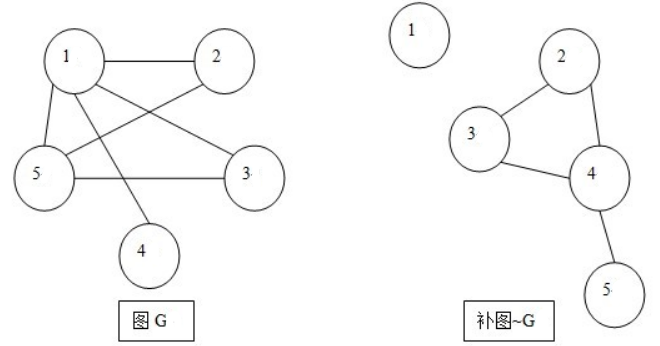# POJ 2942 - Knights of the Round Table

## 问题描述

• 1、 相互憎恨的两个骑士不能坐在直接相邻的2个位置；
• 2、 出席会议的骑士数必须是奇数，这是为了让投票表决议题时都能有结果。

• 1、所给出的憎恨关系一定是双向的，不存在单向憎恨关系。
• 2、由于是圆桌会议，则每个出席的骑士身边必定刚好有2个骑士。即每个骑士的座位两边都必定各有一个骑士。
• 3、一个骑士无法开会，就是说至少有3个骑士才可能开会。

## 解题思路

• 1、补图 的定义
• 2、双连通分量 的定义
• 3、二分图 的定义
• 4、奇圈 的定义
• 5、判定一个图是否为二分图的方法：交叉染色法
• 6、Tarjan算法

1、 补图

2、双连通分量

3、二分图

4、 奇圈

5、交叉染色法判定二分图

6、Tarjan算法

1、 利用m对憎恨对构造图G，则图G中有边相连的两个点表示这2个骑士互相憎恨。

2、 构造图G的补图`~G`，则图`~G`中有边相连的两个点表示这2个骑士可以坐在相邻位置。

3、 在图`~G`中，可能存在某些点的度数 `<=1`，就是说这些骑士旁边最多只能坐另一个骑士，根据圆桌的座位要求每个骑士k的座位两边都必定各有一个骑士（k度数==2），那么我们认为这些度数 `<=1` 的点是孤立的或者是单连通的，也就是说他们不在圆桌的“环”内。4、 现在问题是，我们怎么才能知道哪些骑士在环外？

5、 骑士在双连通分量内（在环内），并不能就此就说明它可以出席会议了，因为假如这个骑士所在的双连通分量，不是一个奇数顶点的环（奇圈），而是一个偶数顶点的环，那么这个双连通分量内的全部骑士都要被亚瑟王开除。

6、 那么怎样判断一个双连通分量是奇圈呢？

• （1）如果一个双连通分量内的某些顶点在一个奇圈中（即双连通分量含有奇圈），那么这个双连通分量的其他顶点也在某个奇圈中；
• （2）如果一个双连通分量含有奇圈，则他必定不是一个二分图。反过来也成立，这是一个充要条件。

7、 显然所有在奇圈中的骑士，都是允许出席会议的，而由于可能有部分骑士允许出席一个以上的会议（即他们是2个以上的奇圈的公共点），那么为了避免重复统计人数，当我们判断出哪些骑士允许出席会议时，就把他们做一个标记（相同的骑士只做一个标记）。最后当Tarjan算法结束后，我们统计一下被标记的人数有多少，再用总人数减去这部分人，剩下的就是被亚瑟王剔除的人数了。

• 1、 为方便输入，图G用邻接矩阵存储，但为了后期处理数据方便，补图`~G`则最好改用邻接链表，图G唯一的作用就只有生成补图`~G`而已。
• 2、 给结点和边都独立开辟一个存储结构。结点是为了链表处理，边是为了Tarjan求双连通分量时的压栈。
• 3、 在弄清楚知识点之前不要盲目做这题，最好先找相关知识点的简单一点的题目做一下练笔。我在Disscuss上发现有同学做这题用了4个月，而我算上做练笔题的时间，前后只用了2天。

## AC 源码

``````//Memory  Time
//632K     2469MS

#include<iostream>
#include<stack>
using namespace std;

/*结点存储结构*/
class Node
{
public:
int id;
class Node* next;
Node():id(0),next(0){}
};

/*边存储结构*/
class Edge
{
public:
Edge(int x=0,int y=0):s(x),t(y){}    //初始化
Edge(const Edge& c):s(c.s),t(c.t){}    //复制

int u(void) const{return s;}
int v(void) const{return t;}

protected:
int s,t;    //边s->t
};

bool operator==(Edge a,Edge b)    //协助函数:==运算符重载
{
return a.u()==b.u() && a.v()==b.v();
}

/*********************************************************/
class solve
{
public:
solve(int n=0,int m=0):N(n),M(m)
{
Initial();
Input();
Struct_G();

for(int i=1;i<=N;i++)    //图G的补图可能不连通
if(!DFN[i])
Tarjan(i,-1);

/*统计留席的骑士数*/
int NotFireNum=0;
for(int j=1;j<=N;j++)
if(NotFire[j])
NotFireNum++;
printf("%d\n",N-NotFireNum); //总数减去留席骑士数，就是被剔除的骑士数
}
~solve()
{
for(int i=1;i<=N;i++)
delete[] G[i];

delete[] Dcc;
delete[] DFN;
delete[] Low;
delete[] NotFire;
delete[] flag;
delete[] color;

EmptyList();

while(!stack_Edge.empty())
stack_Edge.pop();
}

int min(int a,int b) const{return a<b?a:b;}

void Initial(void);                //申请存储空间并初始化
void Input(void);                //输入图G
void Struct_G(void);            //构造图G的补图
void Tarjan(int s,int father);    //Tarjan算法。s:当前搜索位置, father:s的父亲结点
bool IsBinary(int s,int col);    //交叉染色判断s所在的双连通分量是否为二分图(s为搜索起点)

void EmptyList(void);            //清空邻接链表

protected:

int N;                    //the number of Kinghts
int M;                    //the pairs of hate
bool** G;                //邻接矩阵 记录图G

int TimeStamp;            //（外部）时间戳
int* DFN;                //DFN[u]: 结点u的搜索次序（时间戳）
int* Low;                //Low[u]: 结点u或u的子树能够追溯到的最早的栈中结点的次序号

stack<Edge>stack_Edge;    //边栈
int* Dcc;                //存储点的双连通分量
bool* flag;                //标记处于同一个双联通分量的所有顶点
int dNum;                //双连通分量的顶点个数
int* color;                //记录结点所染的颜色
bool* NotFire;            //标记留席的骑士（即没有被剔除的骑士）
};

void solve::Initial(void)
{
/*申请图G的存储空间并初始化*/

G=new bool*[N+1];
for(int i=1;i<=N;i++)
{
G[i]=new bool[N+1];
memset(G[i],false,sizeof(bool)*(N+1));
G[i][i]=true;
}

/*申请图G的补图~G的存储空间并初始化*/

for(int j=0;j<=N;j++)

/*申请执行Tarjan算法所需的存储空间并初始化*/

TimeStamp=0;
DFN=new int[N+1];
Low=new int[N+1];
memset(DFN,0,sizeof(int)*(N+1));
memset(Low,0,sizeof(int)*(N+1));

/*申请用于判定二分图所需的存储空间并初始化*/

Dcc=new int[2*N+1];        //由于是按边压栈，则同一个点可能最多2次入栈
flag=new bool[N+1];
color=new int[N+1];
NotFire=new bool[N+1];
memset(NotFire,false,sizeof(bool)*(N+1));

return;
}

void solve::Input(void)
{
int x,y;        //temporary
for(int j=1;j<=M;j++)
{
scanf("%d %d",&x,&y);
G[x][y]=G[y][x]=true;
}
return;
}

void solve::Struct_G(void)
{
for(int i=1;i<=N;i++)
{
for(int j=1;j<=N;j++)
{
if(!G[i][j])
{
}
}
}
return;
}

void solve::Tarjan(int s,int father)
{
DFN[s]=Low[s]=++TimeStamp;
{
int t=p->id;
if(t!=father && DFN[t]<DFN[s])
{
if(!DFN[t])    //s->t为树枝边
{
Edge tagE(s,t);
stack_Edge.push(tagE);    //树枝边压栈

Tarjan(t,s);
Low[s]=min(Low[s],Low[t]);

if(DFN[s]<=Low[t])        //此时s为割点
{
/*寻找双连通分量，找到一组则马上处理一组*/
dNum=0;
memset(flag,false,sizeof(bool)*(N+1));
memset(color,0,sizeof(int)*(N+1));
for(Edge e=stack_Edge.top();;e=stack_Edge.top())
{
stack_Edge.pop();
Dcc[dNum++]=e.u();
Dcc[dNum++]=e.v();
flag[e.u()]=true;        //标记处于同一个双连通分量的所有点
flag[e.v()]=true;

if(e==tagE || stack_Edge.empty())
break;
}
if(!IsBinary(Dcc,1))        //当前的双连通分量不是二分图，则说明双连通分量内有奇圈
{
for(int i=0;i<dNum;i++)    //奇圈内的所有骑士均留席
NotFire[Dcc[i]]=true;
}
}

}
else        //s->t为后向边
{
Low[s]=min(Low[s],DFN[t]);
}
}
}
return;
}

bool solve::IsBinary(int s,int col)
{
color[s]=col;    //对s染色
{
int t=p->id;
if(flag[t])            //检查t是否与s在同一个双连通分量
{
if(color[t]==0)    //t未染色
{
return IsBinary(t,3-col);    //3-col,目的是轮番用“1、2”交替染色
}
else            //t已染色
{
if(color[s]==color[t])        //若同一条边的两个端点同色
return false;            //说明双连通分量不是二分图
}                                //即双连通分量内有奇圈
}
}
return true;
}

{
if(p->next)
delete p;
return;
}

void solve::EmptyList(void)
{
for(int i=1;i<=N;i++)
return;
}

int main(void)
{
int n,m;
while(scanf("%d %d",&n,&m) && (n+m))
solve poj2942(n,m);

return 0;
}``````

目录